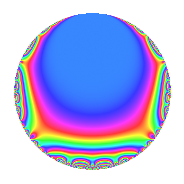# Properties

 Label 2183.1.d.dLevel 2183 Weight 1 Character orbit 2183.d Analytic conductor 1.089 Analytic rank 0 Dimension 2 Projective image $$S_{4}$$ CM/RM No Inner twists 2

# Related objects

## Newspace parameters

 Level: $$N$$ = $$2183 = 37 \cdot 59$$ Weight: $$k$$ = $$1$$ Character orbit: $$[\chi]$$ = 2183.d (of order $$2$$ and degree $$1$$)

## Newform invariants

 Self dual: No Analytic conductor: $$1.08945892258$$ Analytic rank: $$0$$ Dimension: $$2$$ Coefficient field: $$\Q(\sqrt{-2})$$ Coefficient ring: $$\Z[a_1, \ldots, a_{5}]$$ Coefficient ring index: $$1$$ Projective image $$S_{4}$$ Projective field Galois closure of 4.2.2183.1 Artin image size $$48$$ Artin image $\GL(2,3)$ Artin field Galois closure of 8.2.10403062487.1

## $q$-expansion

Coefficients of the $$q$$-expansion are expressed in terms of $$\beta = \sqrt{-2}$$. We also show the integral $$q$$-expansion of the trace form.

 $$f(q)$$ $$=$$ $$q + q^{2} -\beta q^{5} - q^{7} - q^{8} - q^{9} +O(q^{10})$$ $$q + q^{2} -\beta q^{5} - q^{7} - q^{8} - q^{9} -\beta q^{10} + \beta q^{11} - q^{13} - q^{14} - q^{16} -\beta q^{17} - q^{18} + \beta q^{19} + \beta q^{22} - q^{25} - q^{26} -\beta q^{29} - q^{31} -\beta q^{34} + \beta q^{35} + q^{37} + \beta q^{38} + \beta q^{40} - q^{41} + \beta q^{45} -\beta q^{47} - q^{50} - q^{53} + 2 q^{55} + q^{56} -\beta q^{58} - q^{59} + q^{61} - q^{62} + q^{63} + q^{64} + \beta q^{65} + \beta q^{67} + \beta q^{70} - q^{71} + q^{72} -\beta q^{73} + q^{74} -\beta q^{77} + \beta q^{80} + q^{81} - q^{82} -2 q^{85} -\beta q^{88} + q^{89} + \beta q^{90} + q^{91} -\beta q^{94} + 2 q^{95} + q^{97} -\beta q^{99} +O(q^{100})$$ $$\operatorname{Tr}(f)(q)$$ $$=$$ $$2q + 2q^{2} - 2q^{7} - 2q^{8} - 2q^{9} + O(q^{10})$$ $$2q + 2q^{2} - 2q^{7} - 2q^{8} - 2q^{9} - 2q^{13} - 2q^{14} - 2q^{16} - 2q^{18} - 2q^{25} - 2q^{26} - 2q^{31} + 2q^{37} - 2q^{41} - 2q^{50} - 2q^{53} + 4q^{55} + 2q^{56} - 2q^{59} + 2q^{61} - 2q^{62} + 2q^{63} + 2q^{64} - 2q^{71} + 2q^{72} + 2q^{74} + 2q^{81} - 2q^{82} - 4q^{85} + 2q^{89} + 2q^{91} + 4q^{95} + 2q^{97} + O(q^{100})$$

## Character Values

We give the values of $$\chi$$ on generators for $$\left(\mathbb{Z}/2183\mathbb{Z}\right)^\times$$.

 $$n$$ $$297$$ $$1889$$ $$\chi(n)$$ $$-1$$ $$-1$$

## Embeddings

For each embedding $$\iota_m$$ of the coefficient field, the values $$\iota_m(a_n)$$ are shown below.

For more information on an embedded modular form you can click on its label.

Label $$\iota_m(\nu)$$ $$a_{2}$$ $$a_{3}$$ $$a_{4}$$ $$a_{5}$$ $$a_{6}$$ $$a_{7}$$ $$a_{8}$$ $$a_{9}$$ $$a_{10}$$
2182.1
 1.41421i − 1.41421i
1.00000 0 0 1.41421i 0 −1.00000 −1.00000 −1.00000 1.41421i
2182.2 1.00000 0 0 1.41421i 0 −1.00000 −1.00000 −1.00000 1.41421i
 $$n$$: e.g. 2-40 or 990-1000 Significant digits: Format: Complex embeddings Normalized embeddings Satake parameters Satake angles

## Inner twists

Char. orbit Parity Mult. Self Twist Proved
1.a Even 1 trivial yes
2183.d Odd 1 yes

## Hecke kernels

This newform can be constructed as the intersection of the kernels of the following linear operators acting on $$S_{1}^{\mathrm{new}}(2183, [\chi])$$:

 $$T_{2} - 1$$ $$T_{3}$$